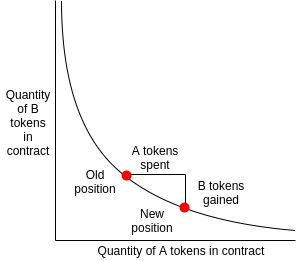# 某島

… : "…アッカリ～ン . .. . " .. .July 2, 2019

## EP 01. Defi 合約鑒賞之 Uniswap（WIP）

[latexpage]

### 做市機制 Market Maker Mechanism

Uniswap 最為突出的特性就是她的做市機制（Market Maker Mechanism）足夠的簡單。與 Bancor 和 P3D 類似，Uniswap 中也沒有傳統交易所中的 Order Book，直接在合約中用市價交易，通過合約中的儲備金來提供流動性。– $x$、$y$ 分別是 transcation 執行前合約中儲備的 ETH 和 DAI。
– $x』$、$y’$ 分別是 transcation 執行結束之後合約中新的的 ETH 和 DAI。
– $\Delta x$ 為下面的 swap() 方法中傳入的 ETH。
– $\Delta y$ 是 swap() 方法兌換出的 DAI。

x*y = k invariant 指的就是：

$$x \times y = k = x』 \times y』 = (x + \Delta x) \times (y + \Delta y)$$

$$Delta y = \frac{k}{(x + \Delta x)} – y$$

eth_pool: uint256
token_pool: uint256

@public
@payable
def ethToTokenSwap():
fee: uint256 = msg.value / 500
invariant: uint256 = self.eth_pool * self.token_pool
new_eth_pool: uint256 = self.eth_pool + msg.value
new_token_pool: uint256 = invariant / (new_eth_pool - fee)
tokens_out: uint256 = self.token_pool - new_token_pool
self.eth_pool = new_eth_pool
self.token_pool = new_token_pool
self.token.transfer(msg.sender, tokens_out


@public
def tokenToEthSwap(tokens_in: uint256):
fee: uint256 = tokens_in / 500
invariant: uint256 = self.eth_pool * self.token_pool
new_token_pool: uint256 = self.token_pool + tokens_in
new_eth_pool: uint256 = self.invariant / (new_token_pool - fee)
eth_out: uint256 = self.eth_pool - new_eth_pool
self.eth_pool = new_eth_pool
self.token_pool = new_token_pool
self.token.transferFrom(msg.sender, self, tokens_out)
send(msg.sender, eth_out)


### 尾聲 Epilogue

（TBD）